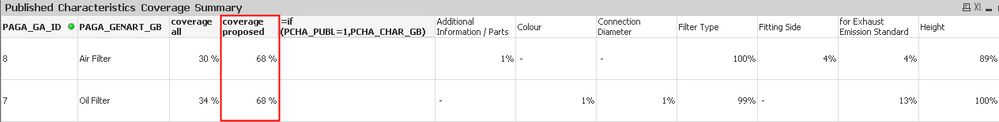# QlikView App Dev

Discussion Board for collaboration related to QlikView App Development.

Announcements
Our next Qlik Insider session will cover new key capabilities. Join us August 11th REGISTER TODAY
cancel
Showing results for
Did you mean:Contributor III

## result of avg() dimension differs from expectation

Dear all,

I have difficulties with the AVG() function.

I created an expression which gives me a coverage

Expression "coverage":

Count({\$<PCHA_VALUE_GB-={'-'}>}PBGA_BG_NO) / max(aggr(nodistinct Count(PBGA_BG_NO), PAGA_GA_ID))

Now I want to calculate the average of this expression as a dimension but the result differs from the total average I can set in the presentation tab:

Dimension "average"

avg(total aggr(Count({\$<PCHA_VALUE_GB-={'-'}>}PBGA_BG_NO) / max(aggr(nodistinct Count(PBGA_BG_NO), PAGA_GA_ID)), PCHA_CHAR_ID, PAGA_GA_ID))chart

Any help is appreciated.

Thanks.

Labels (4)

• ### Total

2 Solutions

Accepted SolutionsMVP

Can you try this

```Avg({<PCHA_PUBL = {1}>} TOTAL Aggr(

Count({\$<PCHA_VALUE_GB -= {'-'}, PCHA_PUBL = {1}>} PBGA_BG_NO)/
Max({<PCHA_PUBL = {1}>}Aggr(NODISTINCT Count({<PCHA_PUBL = {1}>}PBGA_BG_NO), PAGA_GA_ID))

, PCHA_CHAR_ID, PAGA_GA_ID, PCHA_CHAR_GB))```

Or this

```Avg({<PCHA_PUBL = {1}>} TOTAL Aggr(

Count({\$<PCHA_VALUE_GB -= {'-'}>} PBGA_BG_NO)/
Max(Aggr(NODISTINCT Count(PBGA_BG_NO), PAGA_GA_ID))

, PCHA_CHAR_ID, PAGA_GA_ID, PCHA_CHAR_GB))```MVP

Yes it was my bad once again.... remove TOTAL qualifier...

```Aggr(
Avg({<PCHA_PUBL = {1}>} TOTAL Aggr(

Count({\$<PCHA_VALUE_GB -= {'-'}, PCHA_PUBL = {1}>} PBGA_BG_NO)/
Max({<PCHA_PUBL = {1}>}Aggr(NODISTINCT Count({<PCHA_PUBL = {1}>}PBGA_BG_NO), PAGA_GA_ID))

, PCHA_CHAR_ID, PAGA_GA_ID, PCHA_CHAR_GB))
, PAGA_GA_ID)```
18 RepliesMVP

I think you have a third dimension (Characterstic) which you have not added to your Aggr() function. Can you add that and see if that resolves your issue?Contributor III
Author

This is a calculated dimension, however when I include the source of this dimension, no changes are visible:avg(total aggr(Count({\$<PCHA_VALUE_GB-={'-'}>}PBGA_BG_NO) / max(aggr(nodistinct Count(PBGA_BG_NO), PAGA_GA_ID)), PCHA_CHAR_ID, PAGA_GA_ID, PCHA_CHAR_GB))MVP

Can you try this

```Avg({<PCHA_PUBL = {1}>} TOTAL Aggr(

Count({\$<PCHA_VALUE_GB -= {'-'}, PCHA_PUBL = {1}>} PBGA_BG_NO)/
Max({<PCHA_PUBL = {1}>}Aggr(NODISTINCT Count({<PCHA_PUBL = {1}>}PBGA_BG_NO), PAGA_GA_ID))

, PCHA_CHAR_ID, PAGA_GA_ID, PCHA_CHAR_GB))```

Or this

```Avg({<PCHA_PUBL = {1}>} TOTAL Aggr(

Count({\$<PCHA_VALUE_GB -= {'-'}>} PBGA_BG_NO)/
Max(Aggr(NODISTINCT Count(PBGA_BG_NO), PAGA_GA_ID))

, PCHA_CHAR_ID, PAGA_GA_ID, PCHA_CHAR_GB))```Contributor III
Author
Thank you. Both work perfectly.Contributor III
Author

Hi Sunny,

this solution works well when I have only one value in the field PAGA_GA_ID selected.

However when I select more than one it calculates an average of all visible though I need an average for each field PAGA_GA_ID.I tried to amend the aggregation but I failed until now.

=num(
Avg({<PCHA_PUBL={1},PCHA_CHAR_ID = {"=Count(DISTINCT PCHA_CHAR_ID=PCGA_CHAR_ID) > 1"}>}
TOTAL Aggr(
Count({\$<PCHA_VALUE_GB -= {'-'}>} PBGA_BG_NO)
/
Max(Aggr(NODISTINCT Count(PBGA_BG_NO), PAGA_GA_ID))
, PCHA_CHAR_ID, PAGA_GA_ID)),'##0 %')

May you help how I have to adjust the expression to have an average for each PAGA_GA_ID:MVP

Try one of these

```Avg({<PCHA_PUBL = {1}>} TOTAL <PAGA_GA_ID> Aggr(

Count({\$<PCHA_VALUE_GB -= {'-'}, PCHA_PUBL = {1}>} PBGA_BG_NO)/
Max({<PCHA_PUBL = {1}>}Aggr(NODISTINCT Count({<PCHA_PUBL = {1}>}PBGA_BG_NO), PAGA_GA_ID))

, PCHA_CHAR_ID, PAGA_GA_ID, PCHA_CHAR_GB))```

Or this

```Avg({<PCHA_PUBL = {1}>} TOTAL <PAGA_GA_ID> Aggr(

Count({\$<PCHA_VALUE_GB -= {'-'}>} PBGA_BG_NO)/
Max(Aggr(NODISTINCT Count(PBGA_BG_NO), PAGA_GA_ID))

, PCHA_CHAR_ID, PAGA_GA_ID, PCHA_CHAR_GB))```Contributor III
Author
Sadly, they return an "//Error in calculated dimension" though the expression is considered as OK.MVP

When did you switch from using an expression to a calculated dimension? or were you always using this as a calculated dimension?MVP

Give this a try

```Aggr(
Avg({<PCHA_PUBL = {1}>} TOTAL Aggr(

Count({\$<PCHA_VALUE_GB -= {'-'}, PCHA_PUBL = {1}>} PBGA_BG_NO)/
Max({<PCHA_PUBL = {1}>}Aggr(NODISTINCT Count({<PCHA_PUBL = {1}>}PBGA_BG_NO), PAGA_GA_ID))

, PCHA_CHAR_ID, PAGA_GA_ID, PCHA_CHAR_GB))
, PAGA_GA_ID)```Community Browser